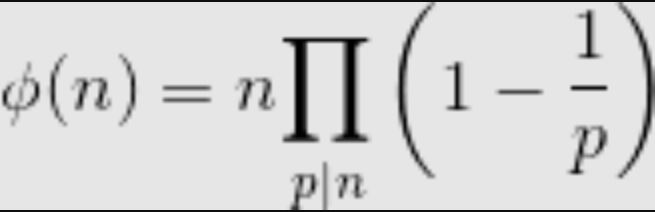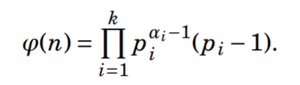Open In App
Related Articles

# Euler’s Totient function for all numbers smaller than or equal to n

Euler’s Totient function Φ(n) for an input n is the count of numbers in {1, 2, 3, …, n} that are relatively prime to n, i.e., the numbers whose GCD (Greatest Common Divisor) with n is 1.

For example, Φ(4) = 2, Φ(3) = 2 and Φ(5) = 4. There are 2 numbers smaller or equal to 4 that are relatively prime to 4, 2 numbers smaller or equal to 3 that are relatively prime to 3. And 4 numbers smaller than or equal to 5 that are relatively prime to 5.
We have discussed different methods for the computation of Φ(n) in the previous post

How to compute Φ for all numbers smaller than or equal to n?

Example:

```Input: n = 5
Output: Totient of 1 is 1
Totient of 2 is 1
Totient of 3 is 2
Totient of 4 is 2
Totient of 5 is 4```

We strongly recommend you to minimize your browser and try this yourself first.
A Simple Solution is to call Φ(i) for i = 1 to n.

An Efficient Solution is to use an idea similar to the Sieve of Eratosthenes to precompute all values. The method is based on below product formula.The formula basically says that the value of Φ(n) is equal to n multiplied by-product of (1 – 1/p) for all prime factors p of n. For example value of Φ(6) = 6 * (1-1/2) * (1 – 1/3) = 2.

Below is the complete algorithm:

```1) Create an array phi[1..n] to store Φ values of all numbers
from 1 to n.

2) Initialize all values such that phi[i] stores i.  This
initialization serves two purposes.
a) To check if phi[i] is already evaluated or not. Note that
the maximum possible phi value of a number i is i-1.
b) To initialize phi[i] as i is multiple in the above product
formula.

3) Run a loop for p = 2 to n
a) If phi[p] is p, means p is not evaluated yet and p is a
prime number (similar to Sieve), otherwise phi[p] must
have been updated in step 3.b
b) Traverse through all multiples of p and update all
multiples of p by multiplying with (1-1/p).

4) Run a loop from i = 1 to n and print all Ph[i] values.```

Below is the implementation of above algorithm.

## C++

 `// C++ program to compute Totient function for``// all numbers smaller than or equal to n.``#include``using` `namespace` `std;` `// Computes and prints totient of all numbers``// smaller than or equal to n.``void` `computeTotient(``int` `n)``{``    ``// Create and initialize an array to store``    ``// phi or totient values``    ``long` `long` `phi[n+1];``    ``for` `(``int` `i=1; i<=n; i++)``        ``phi[i] = i; ``// indicates not evaluated yet``                    ``// and initializes for product``                    ``// formula.` `    ``// Compute other Phi values``    ``for` `(``int` `p=2; p<=n; p++)``    ``{``        ``// If phi[p] is not computed already,``        ``// then number p is prime``        ``if` `(phi[p] == p)``        ``{``            ``// Phi of a prime number p is``            ``// always equal to p-1.``            ``phi[p] = p-1;` `            ``// Update phi values of all``            ``// multiples of p``            ``for` `(``int` `i = 2*p; i<=n; i += p)``            ``{``            ``// Add contribution of p to its``            ``// multiple i by multiplying with``            ``// (1 - 1/p)``            ``phi[i] = (phi[i]/p) * (p-1);``            ``}``        ``}``    ``}` `    ``// Print precomputed phi values``    ``for` `(``int` `i=1; i<=n; i++)``    ``cout << ``"Totient of "` `<< i << ``" is "``        ``<< phi[i] << endl;``}` `// Driver program to test above function``int` `main()``{``    ``int` `n = 12;``    ``computeTotient(n);``    ``return` `0;``}`

## Java

 `// Java program to compute Totient``// function for all numbers smaller``// than or equal to n.``import` `java.util.*;` `class` `GFG {``    ` `// Computes and prints totient of all numbers``// smaller than or equal to n.``static` `void` `computeTotient(``int` `n) {``    ` `    ``// Create and initialize an array to store``    ``// phi or totient values``    ``long` `phi[] = ``new` `long``[n + ``1``];``    ``for` `(``int` `i = ``1``; i <= n; i++)``    ``phi[i] = i; ``// indicates not evaluated yet``                ``// and initializes for product``                ``// formula.` `    ``// Compute other Phi values``    ``for` `(``int` `p = ``2``; p <= n; p++) {``        ` `    ``// If phi[p] is not computed already,``    ``// then number p is prime``    ``if` `(phi[p] == p) {``        ` `        ``// Phi of a prime number p is``        ``// always equal to p-1.``        ``phi[p] = p - ``1``;` `        ``// Update phi values of all``        ``// multiples of p``        ``for` `(``int` `i = ``2` `* p; i <= n; i += p) {``            ` `        ``// Add contribution of p to its``        ``// multiple i by multiplying with``        ``// (1 - 1/p)``        ``phi[i] = (phi[i] / p) * (p - ``1``);``        ``}``    ``}``    ``}` `    ``// Print precomputed phi values``    ``for` `(``int` `i = ``1``; i <= n; i++)``    ``System.out.println(``"Totient of "` `+ i +``                        ``" is "` `+ phi[i]);``}` `// Driver code``public` `static` `void` `main(String[] args) {``    ` `    ``int` `n = ``12``;``    ``computeTotient(n);``}``}` `// This code is contributed by Anant Agarwal.`

## Python3

 `# Python program to compute``# Totient function for``# all numbers smaller than``# or equal to n.` `# Computes and prints``# totient of all numbers``# smaller than or equal to n.``def` `computeTotient(n):` `    ``# Create and initialize``    ``# an array to store``    ``# phi or totient values``    ``phi``=``[]``    ``for` `i ``in` `range``(n ``+` `2``):``        ``phi.append(``0``)` `    ``for` `i ``in` `range``(``1``, n``+``1``):` `        ``phi[i] ``=` `i ``# indicates not evaluated yet``                    ``# and initializes for product``                    ``# formula.` `    ``# Compute other Phi values``    ``for` `p ``in` `range``(``2``,n``+``1``):``    ` `        ``# If phi[p] is not computed already,``        ``# then number p is prime``        ``if` `(phi[p] ``=``=` `p):``        ` `            ``# Phi of a prime number p is``            ``# always equal to p-1.``            ``phi[p] ``=` `p``-``1` `            ``# Update phi values of all``            ``# multiples of p``            ``for` `i ``in` `range``(``2``*``p,n``+``1``,p):``            ` `                ``# Add contribution of p to its``                ``# multiple i by multiplying with``                ``# (1 - 1/p)``                ``phi[i] ``=` `(phi[i]``/``/``p) ``*` `(p``-``1``)``    `  `    ``# Print precomputed phi values``    ``for` `i ``in` `range``(``1``,n``+``1``):``        ``print``(``"Totient of "``, i ,``" is "``,``        ``phi[i])` `# Driver code` `n ``=` `12``computeTotient(n)` `# This code is contributed``# by Anant Agarwal`

## C#

 `// C# program to check if given two``// strings are at distance one.``using` `System;` `class` `GFG``{``    ` `// Computes and prints totient of all``// numbers smaller than or equal to n``static` `void` `computeTotient(``int` `n)``{``    ` `    ``// Create and initialize an array to``    ``// store phi or totient values``    ``long` `[]phi = ``new` `long``[n + 1];``    ``for` `(``int` `i = 1; i <= n; i++)``    ` `    ``// indicates not evaluated yet``    ``// and initializes for product``    ``// formula.``    ``phi[i] = i;``    ` `    ``// Compute other Phi values``    ``for` `(``int` `p = 2; p <= n; p++)``    ``{``        ` `    ``// If phi[p] is not computed already,``    ``// then number p is prime``    ``if` `(phi[p] == p)``    ``{``        ` `        ``// Phi of a prime number p is``        ``// always equal to p-1.``        ``phi[p] = p - 1;` `        ``// Update phi values of all``        ``// multiples of p``        ``for` `(``int` `i = 2 * p; i <= n; i += p)``        ``{``            ` `        ``// Add contribution of p to its``        ``// multiple i by multiplying with``        ``// (1 - 1/p)``        ``phi[i] = (phi[i] / p) * (p - 1);``        ` `        ``}``    ``}``    ``}` `    ``// Print precomputed phi values``    ``for` `(``int` `i = 1; i <= n; i++)``    ``Console.WriteLine(``"Totient of "` `+ i +``" is "` `+ phi[i]);``}` `// Driver code``public` `static` `void` `Main()``{``    ` `    ``int` `n = 12;``    ``computeTotient(n);``}``}` `// This code is contributed by Sam007.`

## PHP

 ``

## Javascript

 ``

Output

```Totient of 1 is 1
Totient of 2 is 1
Totient of 3 is 2
Totient of 4 is 2
Totient of 5 is 4
Totient of 6 is 2
Totient of 7 is 6
Totient of 8 is 4
Totient of 9 is 6
Totient of 10 is 4
Totient of 11 is 10
Totient of 12 is 4```

Time Complexity: O(n2)

Auxiliary Space: O(n)

The same solution can be used when we have a large number of queries for computing the totient function.

Another way to compute Euler’s totient function can also be done by using the below formula:Euler’s totient function

Let us see an example to understand the above function, basically, it does the same job but in a different way:

For example, ϕ(12) = { (2^(2-1)) x (2-1) } x { (3^(1-1)) x (3-1) } =4

Note that ϕ(n) = n−1 if n is prime.

Below is the implementation of the above formula:

## C++

 `// C++ program for the above approach``#include ``using` `namespace` `std;``#define ll long long` `ll Euler_totient_function(ll n)``{``    ``ll result = 1;``    ``for` `(ll i = 2; i * i <= n; i++) {``        ``ll c = 0;``        ``if` `(n % i == 0) {``            ``while` `(n % i == 0) {``                ``c++;``                ``n /= i;``            ``}``        ``}``        ``if` `(c > 0) {``            ``ll power = (ll)``pow``(i, c - 1);``            ``ll sm = (ll)``pow``(i, c - 1) * (i - 1);``            ``result *= sm;``        ``}``    ``}``    ``if` `(n > 1) {``        ``result *= (n - 1);``    ``}``    ``return` `result;``}` `// driver code``int` `main()``{``    ``for` `(ll i = 1; i < 13; i++) {``        ``cout << ``"Euler_totient_function("` `<< i << ``"): "``;``        ``cout << Euler_totient_function(i) << endl;``    ``}``}``#praveeny182`

## Java

 `// Java program for the above approach``import` `java.io.*;` `class` `GFG{` `static` `long` `Euler_totient_function(``long` `n)``{``    ``long` `result = ``1``;``    ``for``(``long` `i = ``2``; i * i <= n; i++)``    ``{``        ``long` `c = ``0``;``        ``if` `(n % i == ``0``)``        ``{``            ``while` `(n % i == ``0``)``            ``{``                ``c++;``                ``n /= i;``            ``}``        ``}``        ``if` `(c > ``0``)``        ``{``            ``long` `power = (``long``)Math.pow(i, c - ``1``);``            ``long` `sm = (``long``)Math.pow(i, c - ``1``) * (i - ``1``);``            ``result *= sm;``        ``}``    ``}``    ``if` `(n > ``1``)``    ``{``        ``result *= (n - ``1``);``    ``}``    ``return` `result;``}` `// Driver code``public` `static` `void` `main(String[] args)``{``    ``for``(``long` `i = ``1``; i < ``13``; i++)``    ``{``        ``System.out.print(``"Euler_totient_function("` `+``                         ``i + ``"): "``);``        ``System.out.println(Euler_totient_function(i));``    ``}``}``}` `// This code is contributed by rishavmahato348`

## Python3

 `# python program for the above approach``import` `math``def` `Euler_totient_function(n):``    ``result ``=` `1``    ``for` `i ``in` `range``(``2``,n``+``1``):``        ``c ``=` `0``        ``if` `n ``%` `i ``=``=` `0``:``            ``while` `(n ``%` `i ``=``=` `0``):``                ``c``+``=``1``                ``n ``/``/``=` `i``        ``if` `(c > ``0``):``            ``power ``=` `math.``pow``(i,c``-``1``)``            ``m ``=` `math.``pow``(i,c``-``1``)``*``(i``-``1``)``            ``result``*``=``m``    ``if` `(n > ``1``):``        ``result ``*``=` `(n ``-` `1``)``    ``return` `int``(result)`  `for` `i ``in` `range``(``1``,``13``):``    ``print``(``"Euler_totient_function("` `, i , ``"): "``,end``=``"")``    ``print``(Euler_totient_function(i))`

## C#

 `// C# program for the above approach``using` `System;` `class` `GFG {` `    ``static` `long` `Euler_totient_function(``long` `n)``    ``{``        ``long` `result = 1;``        ``for` `(``long` `i = 2; i * i <= n; i++) {``            ``long` `c = 0;``            ``if` `(n % i == 0) {``                ``while` `(n % i == 0) {``                    ``c++;``                    ``n /= i;``                ``}``            ``}``            ``if` `(c > 0) {``                ``long` `sm``                    ``= (``long``)Math.Pow(i, c - 1) * (i - 1);``                ``result *= sm;``            ``}``        ``}``        ``if` `(n > 1) {``            ``result *= (n - 1);``        ``}``        ``return` `result;``    ``}` `    ``// Driver code``    ``public` `static` `void` `Main()``    ``{``        ``for` `(``long` `i = 1; i < 13; i++) {``            ``Console.Write(``"Euler_totient_function("` `+ i``                          ``+ ``"): "``);``            ``Console.WriteLine(Euler_totient_function(i));``        ``}``    ``}``}` `// This code is contributed by rishavmahato348`

## Javascript

 ``

Output

```Euler_totient_function(1): 1
Euler_totient_function(2): 1
Euler_totient_function(3): 2
Euler_totient_function(4): 2
Euler_totient_function(5): 4
Euler_totient_function(6): 2
Euler_totient_function(7): 6
Euler_totient_function(8): 4
Euler_totient_function(9): 6
Euler_totient_function(10): 4
Euler_totient_function(11): 10
Euler_totient_function(12): 4```

Time Complexity: O(sqrt(n)*log(n))

Auxiliary Space: O(1)